# Graphing Complex Numbers

in STEMGeeks2 years ago

Hello. In this mathematics post, I cover graphing complex numbers. For those who know some trigonometry, graphing complex numbers is not that much different.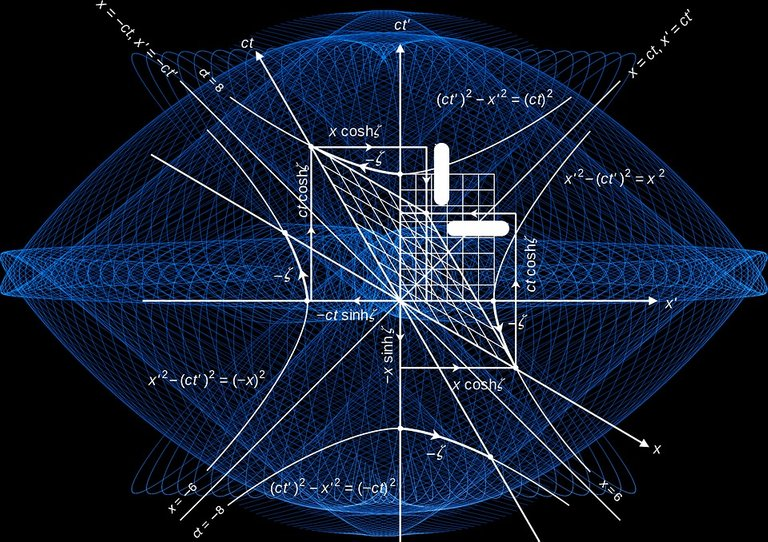Pixabay Image Source

## Real & Imaginary Parts of Complex Numbers

A complex number `z` is of the form: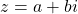where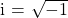, `a` is the real number part of `z` and `b` is the imaginary number part of `z`.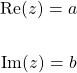## Argand Diagram

A complex number can be represented graphically with the use of a Argand diagram. This diagram is for plotting complex numbers as points. The x-axis is the real axis and the y-axis is the imaginary axis. (Screenshot)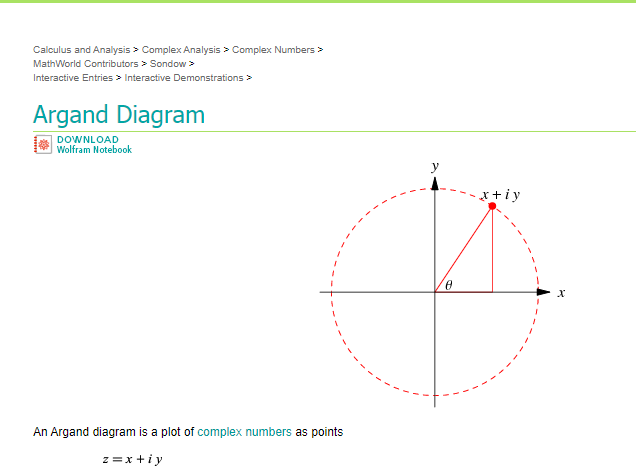Similar to trigonometry with Cartesian co-ordinates and `(x, y)` points, we can find the radius of the point `(a, b)` that represents a complex number. This radius in the complex numbers setting is called the modulus. This modulus is a distance measure that is related to the Pythagorean Theorem. Wolfram uses `z = x + iy` while I use `z = a + bi` in this post.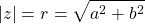Pixabay Image Source

## Theta Angle

After drawing the point for `(a, b)`, we can draw two lines. The first line is the radius from the origin to the point. Line two is a horizontal line from the origin to `(a, 0)`. The angle theta is the angle between these two lines.

Determine The Angle Theta

When the angle theta is unknown, trigonometry is used to find the angle. The tangent of the unknown angle is the opposite side length `b` divided by the adjacent side length of `a`.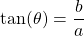Obtaining the angle involves the use of the inverse tangent function (arctan).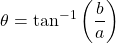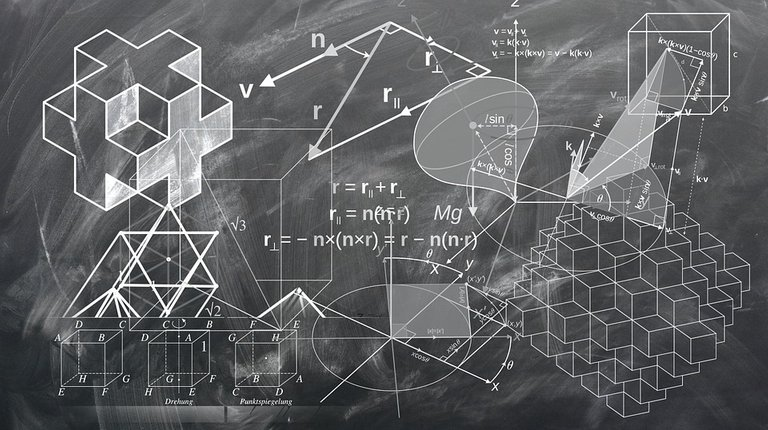Pixabay Image Source

## A Few Examples

Example One

The complex number that we are working with in this example is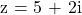. Five is the real part of the complex number and 2 is the imaginary part. The co-ordinate would be (5, 2) on the Argand diagram. (Screenshot with MathisFun drawing website.)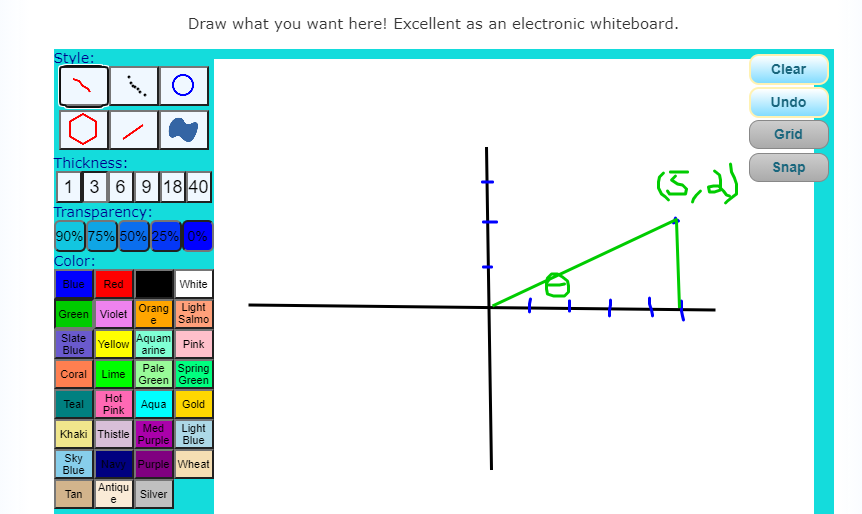The angle associated with the right angled triangle is as follows.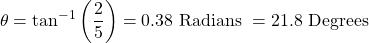Example Two

Consider a different complex number of `z = -2 -4i`. The co-ordinate here is (-2, -4) on the Argand diagram.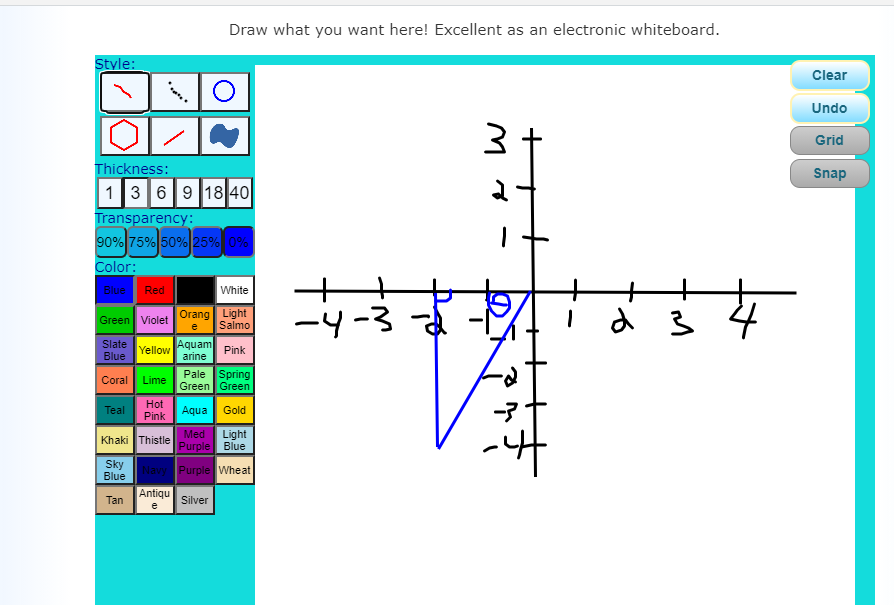Using the formula for finding the angle we have: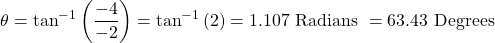The angle theta is for the angle in the triangle. If we want the angle associated with the complex number `z`, we need `arg(z)` which is the angle from the terminal arm to the hypotenuse of the triangle.

In this case, the argument for `z` is 180 degrees + 63.43 degrees which is 243.43 degrees.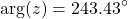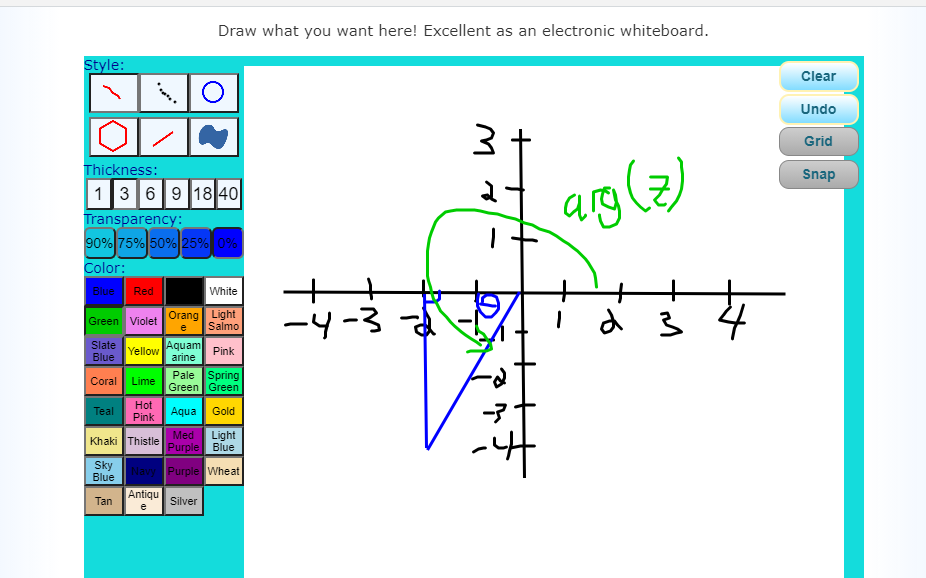Posted with STEMGeeks

Sort:

What a coincidence. I just finished doing complex signal analysis in Matlab when I saw this post. This is a good tutorial for those who are taking ac circuit (especially on phasors).

2 years ago (edited)

Us STEM people have something in common. There are times when we write posts on similar topics.

This post is just based from the math side for complex numbers. I don't know anything on circuits.

There is a follow up post to this that I have prepared.